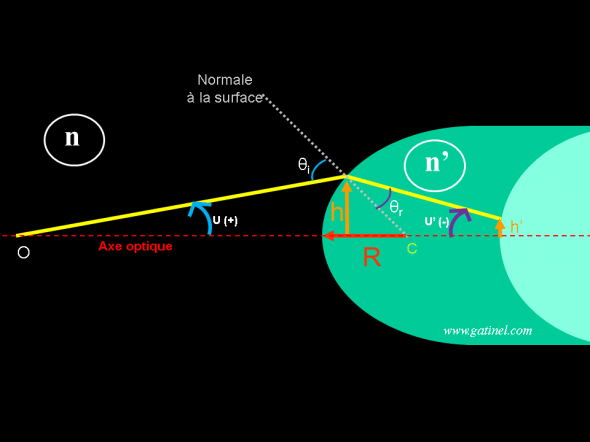+ +

# Paraxial: definitions and explanations

The term paraxial ("close to the axis') is an approximation made in geometrical optics, in order to predict the path of rays of light through an optical system in a simple way. In the paraxial approximation, the angle formed by the incident ray with the optical axis is low (the value of the angle, in radians, is close to that of his sinuses); This allows to perform some geometric simplifications and get forms simple and linear, involving the angle of index and height of the RADIUS at the entrance of the optical system. A paraxial approximation is necessary to establish the formula of vergence. If one knows the vergence of optical system, one can then predict the height and angle of release of the corresponding emerging RADIUS. The paraxial field allows to simplify the calculation of the path of the rays and the formation of pictures through the optical system. This is useful for example to establish the theoretical formulas for the biometric calculation of the power of artificial lens implants.

The non-paraxial field is called marginal area: it is in the field not paraxial optical aberrations effect comes in.

## Eye and conditions paraxiales

Regarding the eye can trace the path of a ray through the front of the cornea, the posterior face of the cornea, etc by a paraxial approximation. Just know the paraxial curvature and the index of refraction of crossed circles. The conditions paraxiales apply for the near rays of the optical axis of the eye: yet, rays admitted by the pupil irisne can form an angle relatively important with this one; for example, for a pupil of 4 mm and a radius of curvature corneal close to 8 mm, the angle of impact i is equal is close to 14.5 ° 0.253 radians. The sine of this angle is 0.250 radians which is more or less acceptable depending on the context, and this especially as the error can be accentuated to each refraction, especially by the cristalliniennes surfaces. In practice, we can make use of for the study paraxial approximation and correction of refractive defects of the eye (ametropias).

In paraxiales conditions, one can equate a set of dioptres or lenses with a system centered, which only requires the homes and plans and main points to determine the properties related to the formation of images (position, magnification, etc.): vergences formulas apply, depending on the position of households and the objects with respect to the principal planes of the / systems.

## Representation

The following diagram represents an incident Ray paraxial refraction, propagating from left to right in a middle of index n. This section forms a u (radians) angle and meets a spherical diopter of RADIUS R, and refractive index, to a height h.The incident rays forming a low angle to the optical axis, to determine the direction of the ray after refraction through the formula didn't u'-n u = h F. This formula stems from the Snell's law applied to small angles. The signs + and - after u and u' depend on the respective direction of these angles, expressed in radians. On the right, shown in light green a possible new refractive surface: simply repeat the calculation with h' (which depends on the distance of the surface from the previous) and u' to determine the path after refraction by the next surface.

We call F the vergence of the diopter, equal to the change of index of refraction (no-n) divided by the radius of curvature R; F = (no-n) / r

In the paraxiales conditions, we check the equation:

don't u'-n u = h F

where F = 1/R (F is expressed in diopters). It is important not to confuse u u' and the angle of incidence, θi and the angle of refraction (see θr the page dedicated to the calculation of the formula of vergence for demonstration This formula)

As suggested in the legend of the previous figure, you can apply the same formula with the next surface: this is done serially in ray tracing calculations.